## Identify Zigzag point for timeseries

Hi Everyone,

I want to find the zigzag point for timeseries and quite surprise, there isn't much discussion on this topic.

In the data below, the zigzag point should be number 10, 1 and 10

The zigzag line is in picture below

Thanks,

HHC

``````data have;
input value;
datalines;
6
7
8
8
10
9
8
8
8
7
6
8
8
4
5
1
2
3
4
6
6
8
9
10
6
8
6
5
1
;run;

data have; set have;
n=_N_;run;``````

1 ACCEPTED SOLUTION

Accepted Solutions

## Re: Identify Zigzag point for timeseries

The responses given so far are quite good. I am not aware of any thing in SAS/ETS or (SAS/ECONOMETRICS) that is specifically designed for peak-discovery.  I think this problem can also be called identifying turning points of a time series.  For noisy time series the interest could be in finding the turning points of the underlying smooth signal.  I will describe a quick way of doing this by using PROC UCM in SAS/ETS.  Of course, this is a solution to a related problem and not the original one.

Let's say the value_t series can be thought of as a sum of  mu_t, a smooth curve, and noise_t: value_t = mu_t + noise_t.  The following code shows you how to fit this model when mu_t is modeled by an integrated random walk, which produces cubic smoothing spline, and Gaussian white noise:

proc ucm data=have;
id n interval=obs;
model value;
irregular;
level variance=0 noest;
slope plot=smooth;
run;

The output data set "for" contains the estimates of mu_t and its slope (the columns s_level and s_slope).  The turning points of mu_t could be located as places where the sign of adjacent s_level values change (i.e., points where s_level stops going upward/downward).  These points are located by the following data steps:

data change;
set for(keep=n value s_level s_slope);
s1 = lag(s_slope);
turn = 0;
if n >= 2 then do;
if sign(s1) ^= sign(s_slope) then turn=1;
end;
run;

*The turns data set contains the discovered turn points: 5 17 24;

data turns;
set change;
where turn=1;
run;

The following plot shows how well s_level approximates the original series: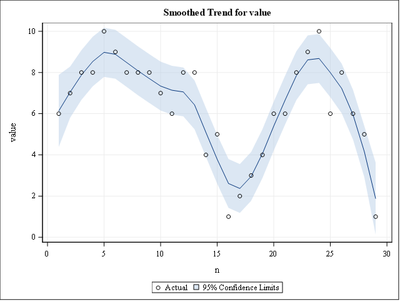The following curve plots the slope (s_slope):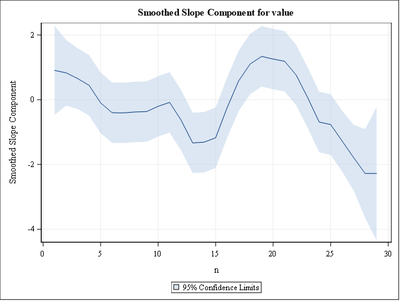The following code shows the discovered turning points:

proc sgplot data=change;
title Plot of smoothed_value with identified turning points;
series x=n y=s_level;
refline 5 17 24/ axis=x;
run;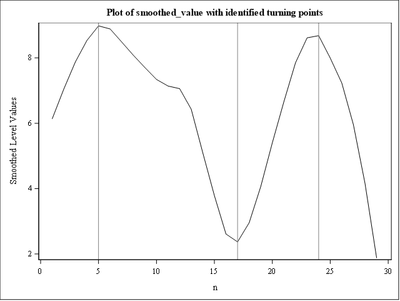Of course, the identified turning points are not the turning points of the original series but of the underlying smooth signal.  The following plot shows how they appear for the original curve:

proc sgplot data=change;
title Plot of value with identified turning points for smoothed_value;
series x=n y=value;
refline 5 17 24/ axis=x;
run;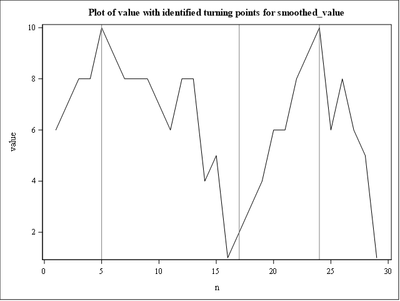I know this is a bit lengthy response.

16 REPLIES 16

## Re: Identify Zigzag point for timeseries

I have never heard of a Zigzag point. What is the logic? And what does your desired result look like here?

Thanks,

HHC

## Re: Identify Zigzag point for timeseries

You want to effectively finds local minima and maxima in a time series.  Those local extremes can then be connected to generate a set of zigzag line segments.

But you have to define for us

1. what "local" is, i.e. how large a window is required to examine for a change in direction, and
2. how large a change in direction must be to designate the local minimum or maximum.
--------------------------
The hash OUTPUT method will overwrite a SAS data set, but not append. That can be costly. Consider voting for Add a HASH object method which would append a hash object to an existing SAS data set

Would enabling PROC SORT to simultaneously output multiple datasets be useful? Then vote for
Allow PROC SORT to output multiple datasets

--------------------------

## Re: Identify Zigzag point for timeseries

@mkeintz , you are 100% correct. The thing is for that Zigzag, normally other software have the "local" and "size of change" parameters and user can adjust.

Can you do something like that?

I find it quite weird that I can't find any code for this very popular timeseries measure 😕

Thanks,

HHCKsharp
Super User

## Re: Identify Zigzag point for timeseries

calling  @Rick_SAS

``````/*Here could give you a start*/
data have;
input value;
n+1;
datalines;
6
7
8
8
10
9
8
8
8
7
6
8
8
4
5
1
2
3
4
6
6
8
9
10
6
8
6
5
1
;

proc iml;
use have;
read all var _num_ into Pts[c=vname];
close;
indices = cvexhull(Pts);            /* compute convex hull of the N points */
hullIdx = indices[loc(indices>0)];
P = Pts[hullIdx, ];
call sort(p,2);
create p from p[c=vname];
append from p;
close;
quit;
data p2;
set p;
sign=sign(dif(value));
run;
data p3;
set p2;
by sign notsorted;
if last.sign;
run;
data want;
set have p3(rename=(n=_n value=_v));
run;

proc sgplot data=want;
series x=n y=value;
scatter x=_n y=_v/markerattrs=(symbol=starfilled color=red);
run;``````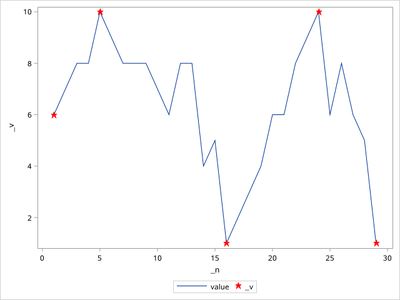## Re: Identify Zigzag point for timeseries

Thank you, Ksharp.

I am digesting your code 😆

HHC

## Re: Identify Zigzag point for timeseries

I haven't heard the term "zigzag point" before, but it seems that you want to identify peaks and valleys in a noisy signal by using certain criteria about the height of the peaks and the width between peaks. In SAS Viya, you can use the PEAKLOC and PEAKINFO functions in SAS IML to identify peaks in a signal. These functions are not available in SAS 9.4.

SAS Event-Stream Processing also supports various methods for locating peaks.

Since this question appears related to time series, I will move it to a more appropriate community where experts like @rselukar might see it and provide additional assistance.Ksharp
Super User

## Re: Identify Zigzag point for timeseries

"In SAS Viya, you can use the PEAKLOC and PEAKINFO functions in SAS IML to identify peaks in a signal. These functions are not available in SAS 9.4. "

Disappointed . Need pay money for it .

## Re: Identify Zigzag point for timeseries

I might have to think about a way to include parameter to the code so I can provide more flexibility.

HHCKsharp
Super User

## Re: Identify Zigzag point for timeseries

You can split this time series into many small series ,and use my code to get these points you want.

Or calling @Rick_SAS ,please let him wrote a IML code for you ?

## Re: Identify Zigzag point for timeseries

LOL. I have a full-time job already, but thanks for thinking of me. Let's hear what the time series experts say.

## Re: Identify Zigzag point for timeseries

The responses given so far are quite good. I am not aware of any thing in SAS/ETS or (SAS/ECONOMETRICS) that is specifically designed for peak-discovery.  I think this problem can also be called identifying turning points of a time series.  For noisy time series the interest could be in finding the turning points of the underlying smooth signal.  I will describe a quick way of doing this by using PROC UCM in SAS/ETS.  Of course, this is a solution to a related problem and not the original one.

Let's say the value_t series can be thought of as a sum of  mu_t, a smooth curve, and noise_t: value_t = mu_t + noise_t.  The following code shows you how to fit this model when mu_t is modeled by an integrated random walk, which produces cubic smoothing spline, and Gaussian white noise:

proc ucm data=have;
id n interval=obs;
model value;
irregular;
level variance=0 noest;
slope plot=smooth;
run;

The output data set "for" contains the estimates of mu_t and its slope (the columns s_level and s_slope).  The turning points of mu_t could be located as places where the sign of adjacent s_level values change (i.e., points where s_level stops going upward/downward).  These points are located by the following data steps:

data change;
set for(keep=n value s_level s_slope);
s1 = lag(s_slope);
turn = 0;
if n >= 2 then do;
if sign(s1) ^= sign(s_slope) then turn=1;
end;
run;

*The turns data set contains the discovered turn points: 5 17 24;

data turns;
set change;
where turn=1;
run;

The following plot shows how well s_level approximates the original series:The following curve plots the slope (s_slope):The following code shows the discovered turning points:

proc sgplot data=change;
title Plot of smoothed_value with identified turning points;
series x=n y=s_level;
refline 5 17 24/ axis=x;
run;Of course, the identified turning points are not the turning points of the original series but of the underlying smooth signal.  The following plot shows how they appear for the original curve:

proc sgplot data=change;
title Plot of value with identified turning points for smoothed_value;
series x=n y=value;
refline 5 17 24/ axis=x;
run;I know this is a bit lengthy response.Ksharp
Super User

## Re: Identify Zigzag point for timeseries

I tested rselukar 's code and got this :

``````data have;
set sashelp.stocks;
if stock='Intel';
keep date stock close;
run;
proc sort data=have;by date;run;
data have2;
set have(keep=close rename=(close=value));
n+1;
run;

proc ucm data=have2;
id n interval=obs;
model value;
irregular;
level variance=0 noest;
slope plot=smooth;
run;

data change;
set for(keep=n value s_level s_slope);
s1 = lag(s_slope);
turn = 0;
if n >= 2 then do;
if sign(s1) ^= sign(s_slope) then turn=1;
end;
run;
data turns;
set change;
where turn=1;
run;
data all;
set have2 turns(keep=n value rename=(n=_n value=_v));
run;
proc sgplot data=all;
title Plot of smoothed_value with identified turning points;
series x=n y=value;
scatter x=_n y=_v/markerattrs=(symbol=starfilled color=red);
run;
``````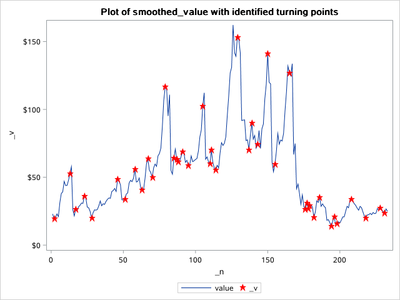Discussion stats
• 16 replies
• 1469 views
• 9 likes
• 6 in conversation